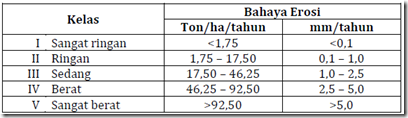Home > English > A Regional Sediment Factors

# A Regional Sediment Factors

As each – each factor can be explained as follows:
A. Erositas Rain (R)
Erosivitas rain is the ability of rainwater as the cause of the erosion that comes from the rate and distribution of raindrops, which both affect the magnitude of the kinetic energy of raindrops. Based on the monthly rainfall data, rainfall erosivitas factor (R).

B. Erodibility Land (K)
The value of soil erodibility (K) is determined by the texture, structure, permeability of soil and organic matter content in the soil (Weschemeier et al, 1971). Determining the value of K can be determined by nomographs or can be calculated by using the equation Hammer, 1970.

C. Slopes (LS)
Map slope obtained from the evaluation of contour lines on a topographic map scale of 1: 50,000 series AMS – T.725 assisted by using the software. In making the value of the index length and slope (LS) is determined only on the slope alone

D. Crop Management (C)
In determining crop management index is determined from a map of land use and land use information on topographic maps or data obtained directly from the field.

E. Soil Conservation (P)
While the determination of soil conservation index is determined on the interpretation species of plants from the land use was evaluated with slope as well as checking on the field.

Erosion Hazard Determination
Erosion hazard is essentially an estimate of the amount of land lost maximum will occur on a unit of land, when crop management and soil conservation has not changed in a long period of time. Soil erosion will be influenced by several factors, including rainfall will affect the erosivitas rainfall, soil erodibility, slope or slope length index, the index of crop management and soil conservation index.

In this case the estimated maximum amount of soil loss that would occur on land unit is calculated by a formula that has been developed by Smith and Wischmeier otherwise known as the Universal Soil Loss Equation (USLE). Calculation of erosion per unit of land is done by riding overlaid factors – factors that affect erosion mentioned above. Then the magnitude of the danger of erosion are classified as shown in Table 7.

Table 7. Erosion Hazard Class"Tasikmalaya, Indonesia"